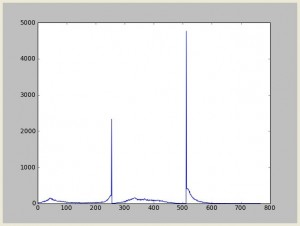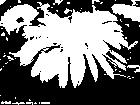# 关于相似图片搜索```对图像Image，记t为前景与背景的分割阈值，前景点数占图像

g=w0*(u0-u)2+w1* (u1-u)2 最大时t即为分割的最佳阈值。
```

1. #coding=utf-8
2. import Image,ImageDraw
3. ret = {}
4. def twoValue(image,G):
5.     for y in xrange(0,image.size):
6.         for x in xrange(0,image.size):
7.             g = image.getpixel((x,y))
8.             if g > G:
9.                 ret[(x,y)] = 1
10.             else:
11.                 ret[(x,y)] = 0
12. def OtsuGray(image):
13.     hist = image.histogram()
14.     totalH = 0
15.     for h in range(0,256):
16.         v =hist[h]
17.         if v == 0 : continue
18.         totalH += v*h
19.     width  = image.size
20.     height = image.size
21.     total  = width*height
22.     v = 0
23.     gMax = 0.0
24.     tIndex = 0
25.     total0 = 0.0
26.     total1 = 0.0
27.     n0H   = 0.0
28.     n1H   = 0.0
29.     for t in range(1,255):
30.         v = hist[t-1]
31.         if v == 0: continue
32.         total0 += v
33.         total1 = total - total0
34.         n0H += (t-1)*v
35.         n1H = totalH - n0H
36.         if total0 > 0 and total1 > 0:
37.             u0 = n0H/total0
38.             u1 = n1H/total1
39.             w0 = total0/total
40.             w1 = 1.0-w0
41.             uD = u0-u1
42.             g = w0 * w1 * uD * uD
43.             if gMax < g:
44.                 gMax   = g
45.                 tIndex = t
46.     return tIndex
47. def saveImage(filename,size):
48.     image = Image.new("1",size)
49.     draw = ImageDraw.Draw(image)
50.     for x in xrange(0,size):
51.         for y in xrange(0,size):
52.             draw.point((x,y),ret[(x,y)])
53.     image.save(filename)
54. def handle(filename,outputname):
55.     im = Image.open(filename)
56.     im = im.convert('L')
57.     otsu = OtsuGray(im)
58.     twoValue(im,otsu)
59.     saveImage(outputname,im.size)
60. if __name__=='__main__':
61.     handle('111.jpg','temp.jpg')ps：话说根据本文的方法你也可以进行验证码识别的一些邪恶举动呢。。。但是不要做坏事哦~

pss：这篇文章介绍一些有趣的图像算法，有兴趣的童鞋可以围观

### 《关于相似图片搜索》有 41 条评论

1.Lingshuang | #1

wr大牛 你是不是心血来潮 开始研究图片搜索啊？ 怎么从来没听你说过啊？

•isnowfy

@Lingshuang: 其实我研究的是验证码识别来着，觉得题目不和谐，换了个名字=。=